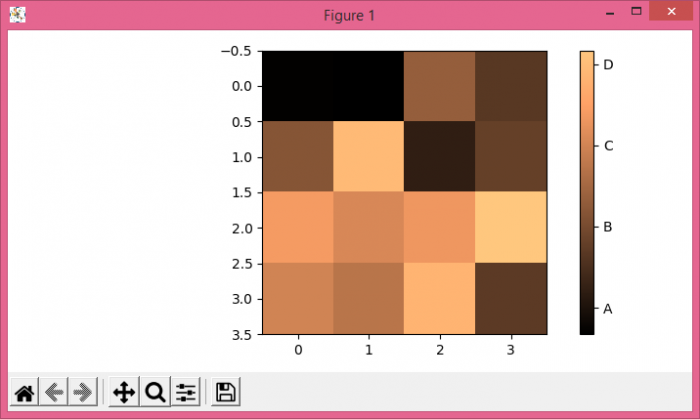# How to set the number of ticks in plt.colorbar in Matplotlib?

To set the number of ticks in a colorbar, we can take the following steps−

• Create random data using numpy
• Display the data as an image, i.e., on a 2D regular raster.
• Make a colorbar using colorbar() method with an image scalar mappable object.
• Set the ticks and tick labels of the colorbar using set_ticks() and set_ticklabels() methods.
• To display the figure, use show() method.

## Example

import numpy as np
from matplotlib import pyplot as plt
plt.rcParams["figure.figsize"] = [7.00, 3.50]
plt.rcParams["figure.autolayout"] = True
data = np.random.rand(4, 4)
im = plt.imshow(data, cmap="copper")
cbar = plt.colorbar(im)
cbar.set_ticks([0.2, 0.4, 0.6, 0.8])
cbar.set_ticklabels(["A", "B", "C", "D"])
plt.show()

## Output